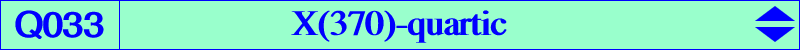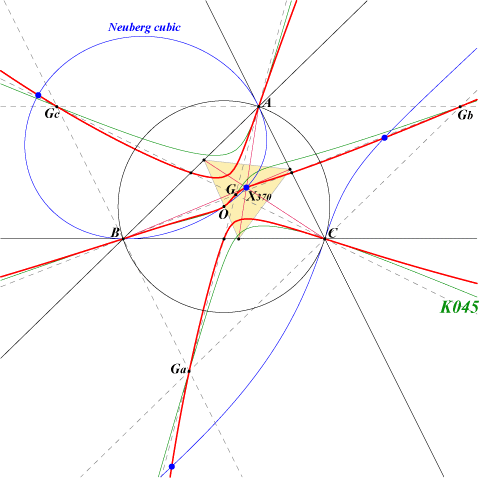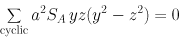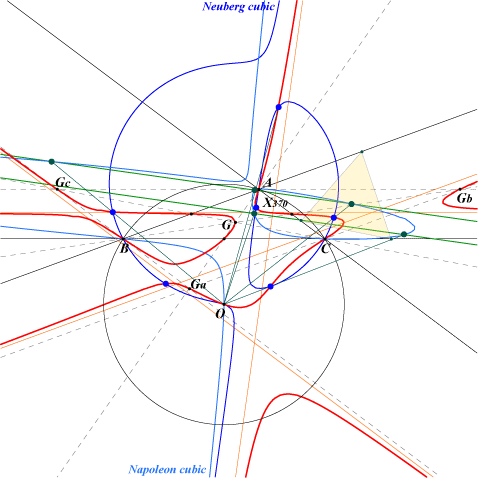X(2), X(3), X(30), X(370) and its five mates*, X(5456) * see Table 10 Ga, Gb, Gc : vertices of the antimedial triangle points at infinity of the sidelines of ABC midpoints of ABCQ033 is the locus of point M such that O, M, the centroid of the cevian triangle of M are collinear. See the related Q034, Q105. It is related to the cubics K143-A-B-C, K144, the Neuberg cubic and the Napoleon cubic. See also K264a, K264b and table 10 : X(370) related curves. More generally, if P is a fixed point, the locus of point M such that P, M, the centroid of the cevian triangle of M are collinear is a similar quartic with equation : ∑ p(y^2 - z^2)yz = 0. See Q045 for example. See also Q099. A construction is given in the page Q002. Other locus properties : Q033 is the locus of point M such that • O, M, tM/M are collinear where tM/M is the cevian quotient of the isotomic conjugate tM of M and M. • M, tM, X264/tM are collinear.Q033 has four real asymptotes (orange lines) : one is parallel to the Euler line and the three other are parallel to the sidelines of ABC. They form a triangle homothetic to ABC at the isogonal conjugate of X(1990), a point on the circum-conic through G and O. The tangents at A, B, C, G, Ga, Gb, Gc pass through O. The third polar of O with respect to Q033 is K045 = pK(X2, X264), the Euler perspector cubic. A, B, C are inflexion points. The common tangent at O to Q033 and the Neuberg cubic is the line X(3)X(54). Q033 contains X(370) and its five mates (blue points). These are the six points having an equilateral cevian triangle : they are not necessarily all real. The centers of these triangles (dark green points) are the intersections of the Napoleon cubic with the perpendicular bisectors of the segments X(13)X(15) and X(14)X(16) (green lines).